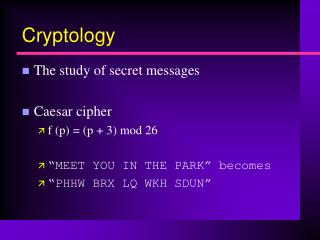# Cryptology - PowerPoint PPT PresentationDownload PresentationCryptology

CryptologyDownload Presentation## Cryptology

- - - - - - - - - - - - - - - - - - - - - - - - - - - E N D - - - - - - - - - - - - - - - - - - - - - - - - - - -
##### Presentation Transcript

1. Cryptology • The study of secret messages • Caesar cipher • f (p) = (p + 3) mod 26 • “MEET YOU IN THE PARK” becomes • “PHHW BRX LQ WKH SDUN”

2. Basic Terminology plaintext - the original message ciphertext - the coded message cipher - algorithm for transforming plaintext to ciphertext key - info used in cipher known only to sender/receiver encipher (encrypt) - converting plaintext to ciphertext decipher (decrypt) - recovering ciphertext from plaintext cryptography - study of encryption principles/methods cryptanalysis (codebreaking) - the study of principles/ methods of deciphering ciphertext without knowing key cryptology - the field of both cryptography and cryptanalysis

3. Letter Pair Frequencies • For instance, given a section of English language, E tends to be very common, while X is very rare. Likewise, ST, NG, TH, and QU are common pairs of letters (termed bigrams or digraphs), while NZ and QJ are rare. The mnemonics phrase "ETAOIN SHRDLU" encodes the 12 most frequent letters in typical English language text.

4. EXCLUSIVE OR The EXCLUSIVE OR is true when exactly one of its operands is true. p q p Å q ____________________ F F F F T T T F T T T F

5. Reversible XOR 1100 plaintext Å 1010 encrypt ----- 0110 ciphertext Å 1010 decrypt ----- 1100 plaintext

6. Reversible XOR 1100 plaintext Å 1010 encrypt Make this value random. ----- 0110 ciphertext Å 1010 decrypt Make this value random. ----- 1100 plaintext

7. Random Numbers • Generating a sequence of random numbers is often useful • In a game, it ensures that a player does not seethe same behavior each time • In a simulation of a complex system,random numbers can be used tohelp generate random events • Car crash in a simulationof a highway system • Likelihood of a gene in cell mutation • Weather simulation

8. Randseed.cpp #include <iostream> #include <string> using namespace std; #include <stdlib.h> #include <time.h> int main() { srand((unsigned int) time(0)); for (int i = 1; i <= 5; ++i) cout << rand() << endl; return 0; }

9. Symmetric Key • Symmetric-key algorithms are a class of algorithms for cryptography that use trivially related, often identical, cryptographic keys for both decryption and encryption.

10. Diffie-Hellman Key Exchange • We both agree on a public key a • I raise ab for some large integer b • You raise ac for integer c • We exchange values • You raise ab to the c power • I raise ac to the b power • We both have abc for our session key (RNG seed) No one eavesdropping can perform log ab or log ac for large values of a, b, c Security Now 34, 7:30mins-11:30

11. RSA Encryption • C = Me mod n • C = encrypted message • M = plaintext message (converted to #s via ASCII) • n is product of two primes pq • e is exponent relatively prime to (p-1)(q-1) (public key) • The formula is invertible if p, q are known • So, huge prime values are chosen for p,q (200 digits) • n cannot be factored in a reasonable amount of time • (2 billion years of computer time) • Receiver of message is given decryption key: p & q • RSA Demo

12. RSA Encryption • The RSA algorithm, invented in 1977 by Ron Rivest, Adi Shamir, and Leonard Adleman, is used frequently for public-key encryption and decryption. • It works as follows: Take two large prime numbers, p and q, and find their product n = pq; n is called the modulus. Choose a number, e, less than n and relatively prime to (p1)(q1), and find d, an inverse of e modulo (p1)(q1), which means that ed 1 mod (p1)(q1); • e and d are called the public and private exponents, respectively. The public key is the pair (n,e); the private key is d. The factors p and q must be kept secret, or destroyed. It is difficult (presumably) to obtain the private key d from the public key (n,e). If one could factor n into p and q (which can be difficult for large values of p and q) however, then one could obtain the private key d. • RSA Demo